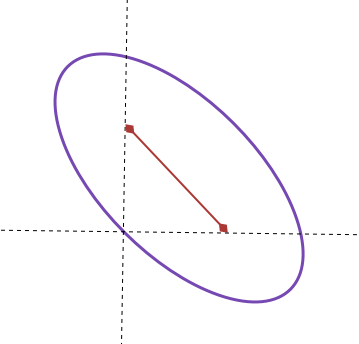# Equation of Ellipse

Algebra Level 5There is an ellipse with focal points

$P = (4, 2)$

and

$Q = (2, 4)$

One of the points on the ellipse is $(2, 2)$. If the equation for this ellipse is

$ax^2 + bxy + cy^2 + dx + ey + f = 0$

where $\gcd(a, b, c, d, e, f) = 1$ find the maximum value of the following expression:

$a + b + c + d + e + f$

×